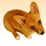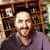# survey123 Date calculation using 12 hour day

227
4
07-24-2020 01:16 AMOccasional Contributor

Hi all,

I am trying work out if it's possible to calculate the 'processing time' we perform during our work day which is 12 hours.

Currently I have questions set up like this:

START_TIME (datetime)

FINISH_TIME (datetime)

DOWNTIME_HRS (decimal)

RUNTIME_HRS (decimal)

The current calculation i have set up is this:

RUNTIME_HRS  =  int(\${FINISH_TIME} - \${START_TIME}) div (1000*60*60) - \${DOWNTIME_HRS}

This works on a 24 hour clock, but doesn't give a true indication of the runtime of the processing as it only operates for 12 hours. So I get incorrect answers if the processing is over 2 days.

For instance, if we are half-way thru processing we will stop at the end of the work day (12 hours), then re-start the next day.

Any idea how I could capture this in the calculation?

Basically looking for a 12 hour day rather than 24 hours.

thanks

Tags (1)
4 RepliesbyEsri Notable Contributor

Hi Tim,

To resolve this I would suggest you need to add more logic into the first part of your calculation, where you minus the start time from finish time. This is where you need to adjust for if the day is different.

My initial thoughts are you could do this with an if statement. So if the day/date is the same for start/finish then simply minus start from finish, but if the day/date is not the same for start/finish then you need to calculate the time for the first day (ie likely 12 hours but may not be) and then calculate the time for the second day, then add them together to get a total over the two days. This can be done in one if statement in the first part of your calculation, or put it separate in other hidden/null questions, and then do the calculation to to convert to hours.

Regards,

Phil.Occasional Contributor

Thanks Philip.

So what I am playing with is this:

START_DAY: format-date(\${START_TIME},'%e') - which gives me an integer for the start DAY

FINISH_DAY: format-date(\${FINISH_TIME},'%e') - which gives me an integer for ending DAY

then,

if(\${START_DAY} = \${FINISH_DAY},int(\${FINISH_TIME} - \${START_TIME}) div (1000*60*60) - \${DOWNTIME_HRS},if(\${FINISH_DAY} - \${START_DAY} = 1,int(\${FINISH_TIME} - \${START_TIME}) div (1000*60*60) -12 - \${DOWNTIME_HRS}))....and so on depending on the equation FINISH_DAY - START_DAY

This doesn't work though if the dates go from 31st July to the 1st August.

Is there a format-date calc that will give me a whole number for a 'date' ?

e.g. can I convert 31/07/2020 to a 'number' ?byEsri Notable Contributor

Hi Tim,

Looks like you are on the right track, but the change over to a new month may cause an issue based on how you have done your calculation. I am not aware if a format-date calc to give a whole number as you asked, so you may need to build in more logic, or use a previous hidden question etc, to work out the if the months are the same, there use different calculations if they are different.

Regards,

Phil.Occasional Contributor

righto....think I sorted it out.

I created a new question - round(decimal-date-time... for both the start and end time/dates.

This generates a number which i then used for my conditional calculation.

If end date - start date = 1, then...

If end date - start date = 2, then...

If end date - start date = 3, then...

and so forth...

thanks